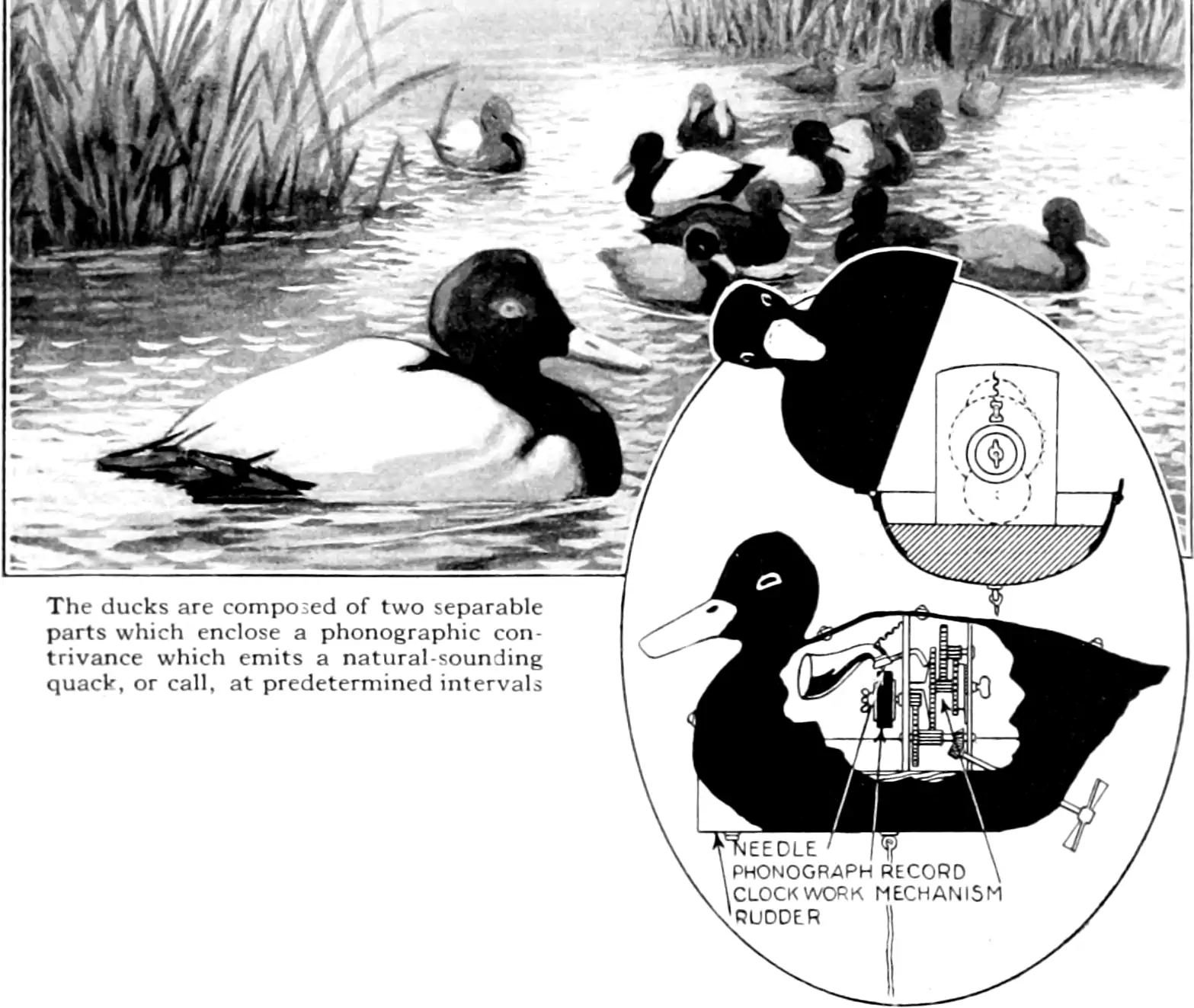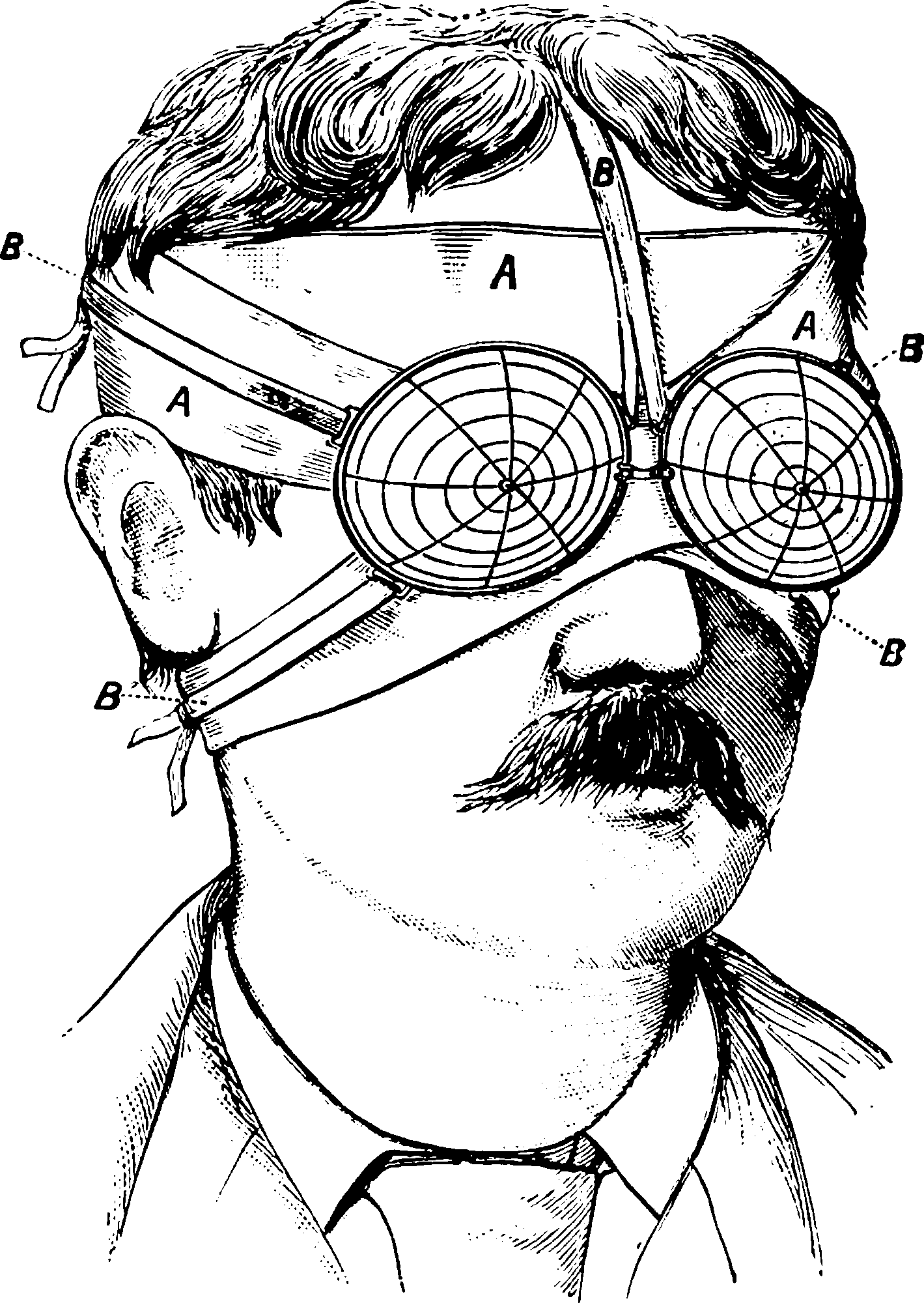# Mind as statistical learnerVarious morsels on the theme of what-machine-learning-teaches-us-about-our-own-learning. Thus biomimetic algorithms find their converse in our algo-mimetic biology.

This should be more about general learning theory insights. Nitty gritty details about how computing is done by biological systems is more what I think of as biocomputing. If you can unify those then well done, you can grow minds in a petri dish.

Eliezer Yudkowsky’s essay, How an algorithm feels from the inside.

## Language theory

The OG test-case of mind-like behaviour is grammatical inference, where a lot of ink was spilled over learnability of languages of various kinds. This is less popular nowadays, where Natural language processing by computer is doing rather interesting things without bothering with the details of formal syntax or traditional semantics. What does that mean? I do not hazard opinions on that because I am too busy for now to form them.

## Descriptive statistical models of cognitionSee, e.g. a Bayesian model of human problem-solving, Probabilistic Models of Cognition, by Noah Goodman and Joshua Tenenbaum and others, which is also a probabilitic programming textbook.

This book explores the probabilistic approach to cognitive science, which models learning and reasoning as inference in complex probabilistic models. We examine how a broad range of empirical phenomena, including intuitive physics, concept learning, causal reasoning, social cognition, and language understanding, can be modeled using probabilistic programs (using the WebPPL language).

Disclaimer, I have not actually read the book.

This descriptive model is not the same thing as the normative model of Bayesian cognition.

do something different again, finding ML models that are good “second-order” fits to how people seem to learn things in practice.

## That free energy thing

This section is dedicated to vivisecting a confusing discussion happening in the literature which I have not looked in to. It is could be a profound insight, or terminological confusion, or a re-statement of the Bayes brain stuff with weirder prose style. I may return one day and decide which.

In this realm, the “free energy principle” is instrumental as a unifying concept for learning systems such as brains.

Here is the most compact version I could find:

The free energy principle (FEP) claims that self-organization in biological agents is driven by variational free energy (FE) minimization in a generative probabilistic model of the agent’s environment.

The chief pusher of this wheelbarrow appears to be Karl Friston.

He starts his Nature Reviews Neuroscience with this statement of the principle:

The free-energy principle says that any self-organizing system that is at equilibrium with its environment must minimize its free energy.

Is that “must” in the sense that it is a

• moral obligation, or
• a testable conservation law of some kind?

If the latter, self-organising in what sense? What type of equilibrium? For which definition of the free energy? What is our chief experimental evidence for this hypothesis?

I think it means that any right thinking brain, seeking to avoid the vice of slothful and decadent perception after the manner of foreigners and compulsive masturbators, would do well to seek to maximise its free energy before partaking of a stimulating and refreshing physical recreation such as a game of cricket.

We do get a definition of free energy itself, with a diagram, which

…shows the dependencies among the quantities that define free energy. These include the internal states of the brain $$\mu(t)$$ and quantities describing its exchange with the environment: sensory signals (and their motion) $$\bar{s}(t) = [s,s',s''…]^T$$ plus action $$a(t)$$. The environment is described by equations of motion, which specify the trajectory of its hidden states. The causes $$\vartheta \supset {\bar{x}, \theta, \gamma }$$ of sensory input comprise hidden states $$\bar{x} (t),$$ parameters $$\theta$$, and precisions $$\gamma$$ controlling the amplitude of the random fluctuations $$\bar{z}(t)$$ and $$\bar{w}(t)$$. Internal brain states and action minimize free energy $$F(\bar{s}, \mu)$$, which is a function of sensory input and a probabilistic representation $$q(\vartheta|\mu)$$ of its causes. This representation is called the recognition density and is encoded by internal states $$\mu$$.

The free energy depends on two probability densities: the recognition density $$q(\vartheta|\mu)$$ and one that generates sensory samples and their causes, $$p(\bar{s},\vartheta|m)$$. The latter represents a probabilistic generative model (denoted by $$m$$), the form of which is entailed by the agent or brain…

$F = -<\ln p(\bar{s},\vartheta|m)>_q + -<\ln q(\vartheta|\mu)>_q$

This is (minus the actions) the variational free energy principle in Bayesian inference.

OK, so self-organising systems must improve their variational approximations to posterior beliefs? What is the contentful prediction here?

See also: the Slate Star Codex Friston dogpile, based on an exposition by Wolfgang Schwarz.

### No comments yet. Why not leave one?

GitHub-flavored Markdown & a sane subset of HTML is supported.